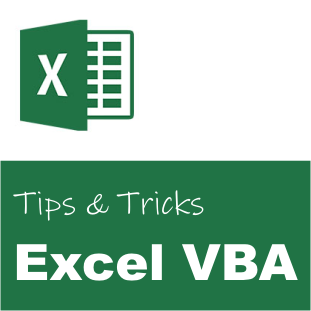# Excel VBA: Convert Column Index to Column Name# Excel VBA: Convert Column Index to Column Name

This article demonstrates how you can convert a Excel column index to the corresponding alphabetical character name of the column. For example, the column number 30 will be converted to the equivalent string “AD”.

I often use this function when I am referring to ranges in Excel, or when I dynamically generate formulas.

```' Column Index To Column Name
Public Function ColumnIndexToColumnName(ByVal intColumnIndex As Integer) As String
' Constants
Const NUMBER_BASE As Integer = 26   ' 26th number system, i.e. A-Z
Const MAX_COLUMNS As Integer = 256
Const ASCII_A As Integer = 65

' Variables
Dim strName As String
Dim strChar As String
Dim intValue As Integer

' Valid index?
If (intColumnIndex < 1) Then
' Error
Err.Raise 234, , "The column index can not be less than 1!"
Exit Function
End If

' Valid index?
If (intColumnIndex > MAX_COLUMNS) Then
' Error
Err.Raise 234, , "The column index can not be more than " & MAX_COLUMNS & "!"
Exit Function
End If

' Build string based on the 26th numbering system,
' i.e. A-Z

' Build name string
Do While (intColumnIndex > 0)
' Get the remaining value
intValue = ((intColumnIndex - 1) Mod NUMBER_BASE)

' Remove handled value
intColumnIndex = (intColumnIndex - 1) \ NUMBER_BASE

' Get the character
strChar = Chr(intValue + ASCII_A)

' Build the name
strName = strChar & strName
Loop

' Return the name
ColumnIndexToColumnName = strName
End Function```

### Usage

If you would like to dynamically generate a formula with cell references in Excel, it doesn’t work to specify a column index. If you know the row- and column index, then you can generate the valid Excel cell reference name.

```' Example constants
Const ROW_INDEX As Integer = 50
Const COLUMN_INDEX As Integer = 30

' Result
Dim strReference As String
Dim objRange As Range

' Convert row index 50 and column index 30 to a valid cell reference, i.e. AD50
strReference = ColumnIndexToColumnName(COLUMN_INDEX) & CStr(ROW_INDEX)

' Get the single cell range
Set objRange = Range(strReference)```

### Related##### Ulf Emsoy
Ulf Emsoy has long working experience in project management, software development and supply chain management.